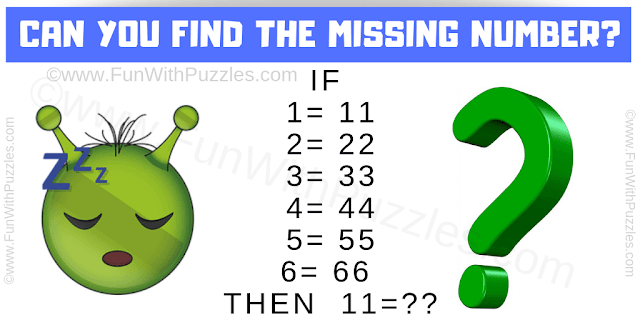This is a fun #maths #logic #question which will trick your mind. More than 90% of people will fail to answer this math logical question correctly. In the given puzzle picture, you are shown some number equations. You have to study these given number equation and then find the missing number which replaces question mark in the last equation.Can you solve this Maths Logical Question?

Answer of this "Maths Logical Question", can be viewed by clicking on the answer button.